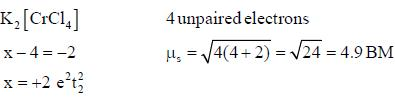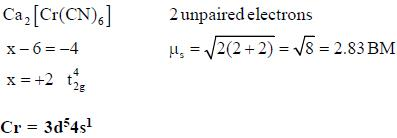Courses

# Transition Metals & Coordination Compounds

## 20 Questions MCQ Test GATE Chemistry Mock Test Series | Transition Metals & Coordination Compounds

Description
This mock test of Transition Metals & Coordination Compounds for Chemistry helps you for every Chemistry entrance exam. This contains 20 Multiple Choice Questions for Chemistry Transition Metals & Coordination Compounds (mcq) to study with solutions a complete question bank. The solved questions answers in this Transition Metals & Coordination Compounds quiz give you a good mix of easy questions and tough questions. Chemistry students definitely take this Transition Metals & Coordination Compounds exercise for a better result in the exam. You can find other Transition Metals & Coordination Compounds extra questions, long questions & short questions for Chemistry on EduRev as well by searching above.
QUESTION: 1

### The ligand which can act as an ambidentate ligands in its complexes is

Solution: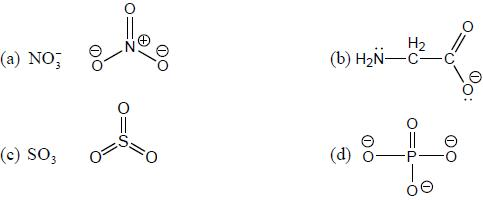There is free electron present on N and O atoms inNH2CH2COO- Therefore, NH2CH2COO- is behave as ambidentate ligand.

QUESTION: 2

### Which one of the following lias the lowest value of magnetic moment?

Solution: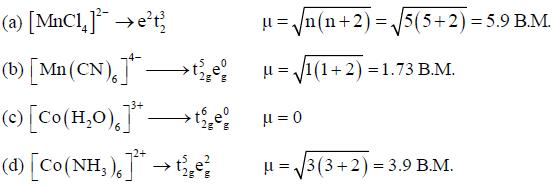QUESTION: 3

### The magnetic moment of Cr2+ (gas) can be calculated by using the formula

Solution:

No quenching o f orbital m otion in Cr2+(gas). its magnetic moment is calculated by the fomiula.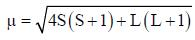QUESTION: 4

The complex which absorbs lowest energy for its transition is

Solution:

In all the complexes the oxidation state of Cr is +3. Tlie splitting of d-orbitals increases with increasing ligand field strength. Since. CT is the weak ligand,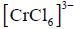shows lowest energy transition

QUESTION: 5

The π-acidity order of the following ligands is

Solution:

π -acidity order ( π -acceptor) is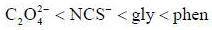QUESTION: 6

Which of the following complexes shows orbital contribution to the magnetic moment?

Solution: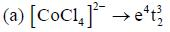Since, e and t2 both are symmetrically filled, there is no orbital contribution.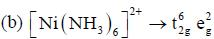Since, t2g and eg both symmetrically filled → no orbital contribution.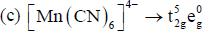Since, t2g unsymmetrically filled → orbital contribution.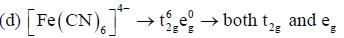Symmetrically filled → no orbital contribution

*Answer can only contain numeric values
QUESTION: 7

The crystal field splitting energy value_________cm-1, for [Ti(h2O)6]+3 that has an absorption maximum at 492 nm.

Solution: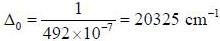*Answer can only contain numeric values
QUESTION: 8

Crystal field splitting energy Δ0 for [ Cr (NH3)6]2+ is 10200 cm-1. The Δt for [Cr(NH3)4]2+ is ___________cm-1.

Solution: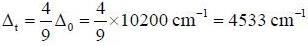*Answer can only contain numeric values
QUESTION: 9

The number of spinels having equal number of A2+ and B3+ ions in octahedral and tetrahedral voids are________
NiCr2O4, FeCr2O4, CO3O4, CrFe2O4, AlMg2O4, NiGa2O4

Solution:

The inverse spinel has the equal number of A2+ and b3+ ions in octahedral voids.

*Answer can only contain numeric values
QUESTION: 10

The number of complexes shows orbital contribution to the magnetic moment are:_________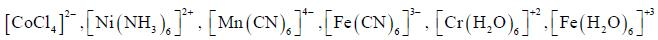Solution:

The complexes in which t2 group orbitals are unsymme trie ally filled, show orbital contribution to the magnetic moment

QUESTION: 11

Which one of the following pair of complexes shows equal number of stereoisomers

Solution: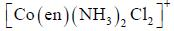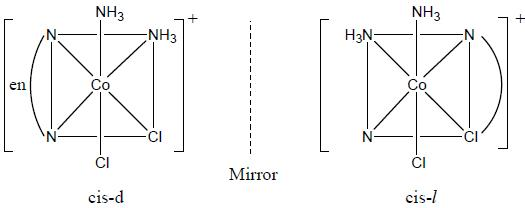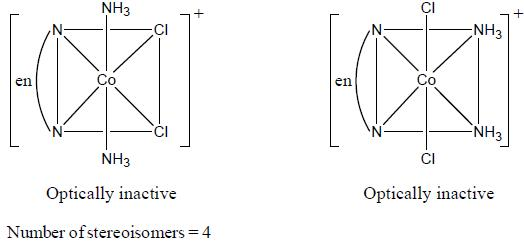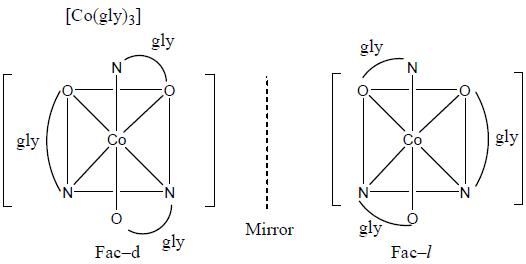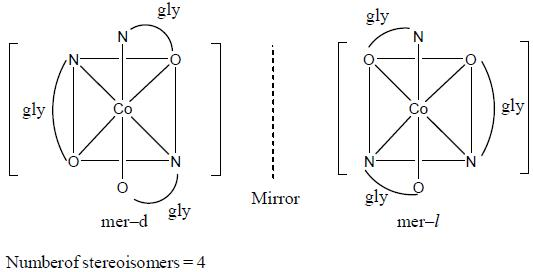QUESTION: 12

The increasing order of inertness of the following complexes is

Solution:

Higher the loss in CFSE, higher will the inertness.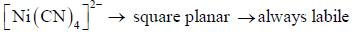Loss in CFSE for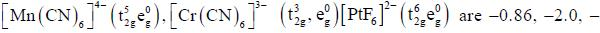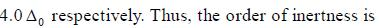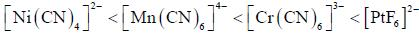QUESTION: 13

According to molecular orbital theory, the electronic configuration of [CoC14]2- ion is

Solution: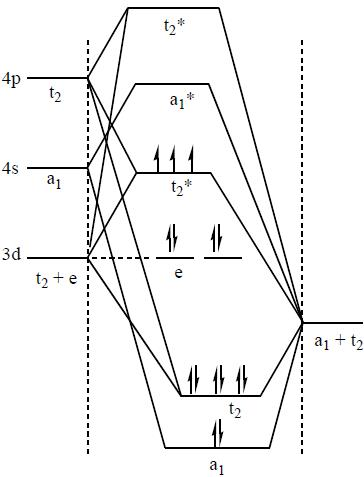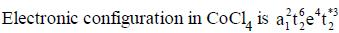QUESTION: 14

Predict tlie products (X) and (Y) in the following reaction: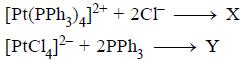Solution: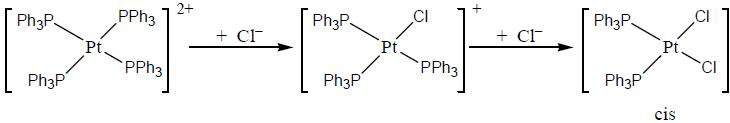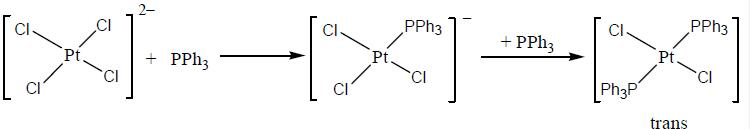QUESTION: 15

Consider the following reaction sequence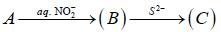Conect set regarding A, B are C is

Solution: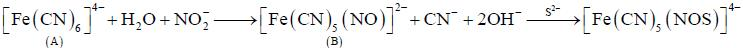QUESTION: 16

Treatment of alkaline solution of A with 30% H2O2 , leads to formation of red colour complex B. The The correct set regarding A and B is /are

Solution: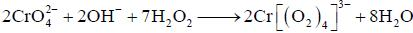QUESTION: 17

The rate of electron transfer in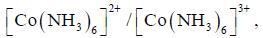system compare to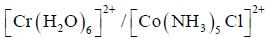system is

Solution: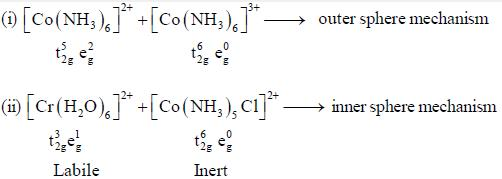In reaction (ii). there is a briding ligand present with makes the reaction more feasible than (i) Thus, electron transfer in (ii) reaction occurs taster than (i)

*Answer can only contain numeric values
QUESTION: 18

The number of complexes among the following show optical isomerism are_______________.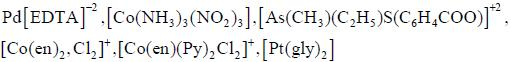Solution: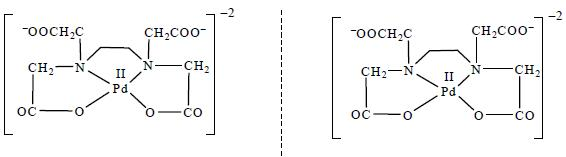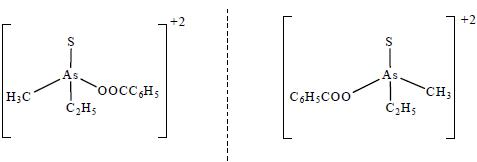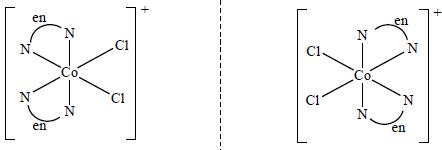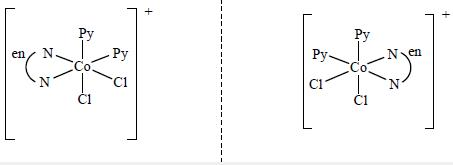*Answer can only contain numeric values
QUESTION: 19

Number of micro states for 3p13 d1 configuration is_________________

Solution:

Number of microstates =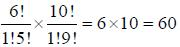*Answer can only contain numeric values
QUESTION: 20

The following complexes K2[MC14]J and C a[M( CN )6] have spin only magnetic moment o f 4.9 BM and 2.83 BM. The electrons present in valence shell d-orbital of neutral gaseous atom of M are______.

Solution: